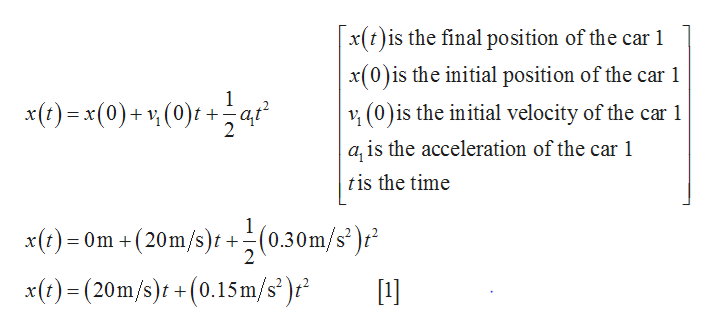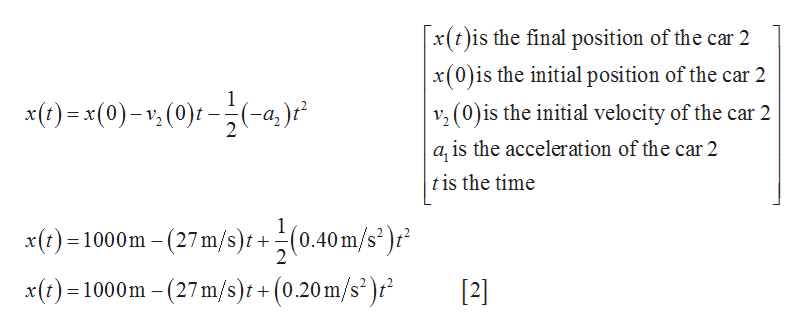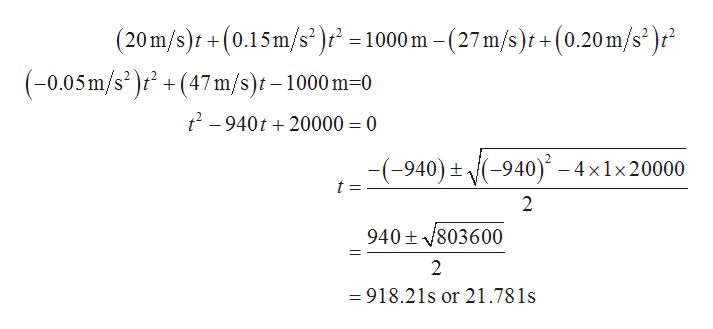# Two cars drive on a straight highway. At time t=0, car 1 passes mile marker 0 traveling due east with a speed of 20.0 m/s . At the same time, car 2 is 1.0 km east of mile marker 0 traveling at 27.0 m/s due west. Car 1 is speeding up with an acceleration of magnitude 0.30 m/s2 , and car 2 is slowing down with an acceleration of magnitude 0.40 m/s2 .At what time do the cars pass next to one another?

Question
148 views

Two cars drive on a straight highway. At time t=0, car 1 passes mile marker 0 traveling due east with a speed of 20.0 m/s . At the same time, car 2 is 1.0 km east of mile marker 0 traveling at 27.0 m/s due west. Car 1 is speeding up with an acceleration of magnitude 0.30 m/s2 , and car 2 is slowing down with an acceleration of magnitude 0.40 m/s2 .

At what time do the cars pass next to one another?

check_circle

Step 1

Write the equation of motion for car 1 and substitute 20m/s for v1(0), and 0.30m/s2 for a1 in the below equation.help_outlineImage Transcriptionclosex(t)is the final position of the car 1 x(0)is the initial position of the car 1 (0)is the initial velocity of the car 1 a, is the acceleration of the car 1 1 x()x(0)+ (0)at 2 tis the time 1 x) Om+ (20m/s)r +(0.30m/s) x(t)(20m/s) +(0.15m/s" )r 2  fullscreen
Step 2

Write the equation of motion for car 2 and substitute 27m/s for v2(0), and 0.40m/s2 for a2 in the below equation.help_outlineImage Transcriptionclosex(t)is the final position of the car 2 x(0)is the initial position of the car 2 v (0) s the initial velocity of the car 2 1 x(t)x(0)-(0)(-4) 2 a, is the acceleration of the car 2 t is the time 1 x(t)1000m-(27m/s)t +(0.40m/s )r 2 = 2 x()1000m -(27m/s)t + (0.20m/s2)'  fullscreen
Step 3

Since the final position of the cars will be same as they pass each other, thus Equat...help_outlineImage Transcriptionclose(20m/s)t+(0.15m/s2)t =1000 m -(27m/s)t+ (0.20m/s)r (-0.05m/s2 (47m/s) 1000 m-0 2-940t20000 = 0 (-940)-4x1x20000 -(-940) t = 2 940 803600 2 = 918.21s or 21.781s fullscreen

### Want to see the full answer?

See Solution

#### Want to see this answer and more?

Solutions are written by subject experts who are available 24/7. Questions are typically answered within 1 hour.*

See Solution
*Response times may vary by subject and question.
Tagged in

### Kinematics